### OUTPUT Statement

OUTPUT <OUT=SAS-data-set> <keyword=name> …<keyword=name> ;

The OUTPUT statement creates a new SAS data set containing statistics calculated after fitting the model. At least one specification of the form keyword=name is required.

All variables in the original data set are included in the new data set, along with the variables created as options for the OUTPUT statement. These new variables contain fitted values and estimated quantiles. If you want to create a SAS data set in a permanent library, you must specify a two-level name. For more information about permanent libraries and SAS data sets, see SAS Language Reference: Concepts. Each OUTPUT statement applies to the preceding MODEL statement. See Example 51.1 for illustrations of the OUTPUT statement.

The following specifications can appear in the OUTPUT statement:

OUT=SAS-data-set

specifies the new data set. By default, the procedure uses the DATAn convention to name the new data set.

keyword=name

specifies the statistics to include in the output data set and gives names to the new variables. Specify a keyword for each desired statistic (see the following list of keywords), an equal sign, and the variable to contain the statistic.

The keywords allowed and the statistics they represent are as follows:

CENSORED=variable

specifies a variable to signal whether an observation is censored, and the type of censoring. The variable takes on values according to Table 51.10.

Table 51.10: Censoring Variable Values

Type of Response

CENSORED Variable Value

Uncensored

0

Right-censored

1

Left-censored

2

Interval-censored

3

CDF=variable

specifies a variable to contain the estimates of the cumulative distribution function evaluated at the observed response. If the data are interval censored, then the cumulative distribution function is evaluated at the response lower interval endpoint. See the section Predicted Values for more information.

CONTROL=variable

specifies a variable in the input data set to control the estimation of quantiles. See Example 51.1 for an illustration. If the specified variable has the value 1, estimates for all the values listed in the QUANTILE= list are computed for that observation in the input data set; otherwise, no estimates are computed. If no CONTROL= variable is specified, all quantiles are estimated for all observations. If the response variable in the MODEL statement is binomial, then this option has no effect.

CRESIDUAL | CRES=variable

specifies a variable to contain the Cox-Snell residuals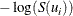where S is the standard survival function and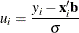If the data are interval censored, residuals are computed for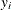values corresponding to lower interval endpoints. If the response variable in the corresponding model statement is binomial, then the residuals are not computed, and this variable contains missing values.

SRESIDUAL | SRES=variable

specifies a variable to contain the standardized residuals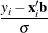If the data are interval censored, residuals are computed forvalues corresponding to lower interval endpoints. If the response variable in the corresponding model statement is binomial, then the residuals are not computed, and this variable contains missing values.

PREDICTED | P=variable

specifies a variable to contain the quantile estimates. If the response variable in the corresponding model statement is binomial, then this variable contains the estimated probabilities,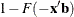.

QUANTILES | QUANTILE | Q=value-list

gives a list of values for which quantiles are calculated. The values must be between 0 and 1, noninclusive. For each value, a corresponding quantile is estimated. This option is not used if the response variable in the corresponding MODEL statement is binomial.

By default, QUANTILES=0.5. When the response is not binomial, a numeric variable, _PROB_, is added to the OUTPUT data set whenever the QUANTILES= option is specified. The variable _PROB_ gives the probability value for the quantile estimates. These are the values taken from the QUANTILES= list and are given as values between 0 and 1, not as values between 0 and 100. The list of QUANTILES values can be specified as in Table 51.11.

Table 51.11: Types of Value Lists

Type of List

Specification

List separated by blanks

.2 .4 .6 .8

List separated by commas

.2,.4,.6,.8

x to y

.2 to .8

x to y by z

.2 to .8 by .1

Combination of methods

.1,.2 to .8 by .2

STD_ERR | STD=variable

specifies a variable to contain the estimates of the standard errors of the estimated quantiles or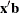. If the response used in the MODEL statement is a binomial response, then these are the standard errors of. Otherwise, they are the standard errors of the quantile estimates. These estimates can be used to compute confidence intervals for the quantiles. However, if the model is fit to the log of the event time, better confidence intervals can usually be computed by transforming the confidence intervals for the log response. See Example 51.1 for such a transformation.

XBETA=variable

specifies a variable to contain the computed value of, whereis the covariate vector and b is the vector of parameter estimates.